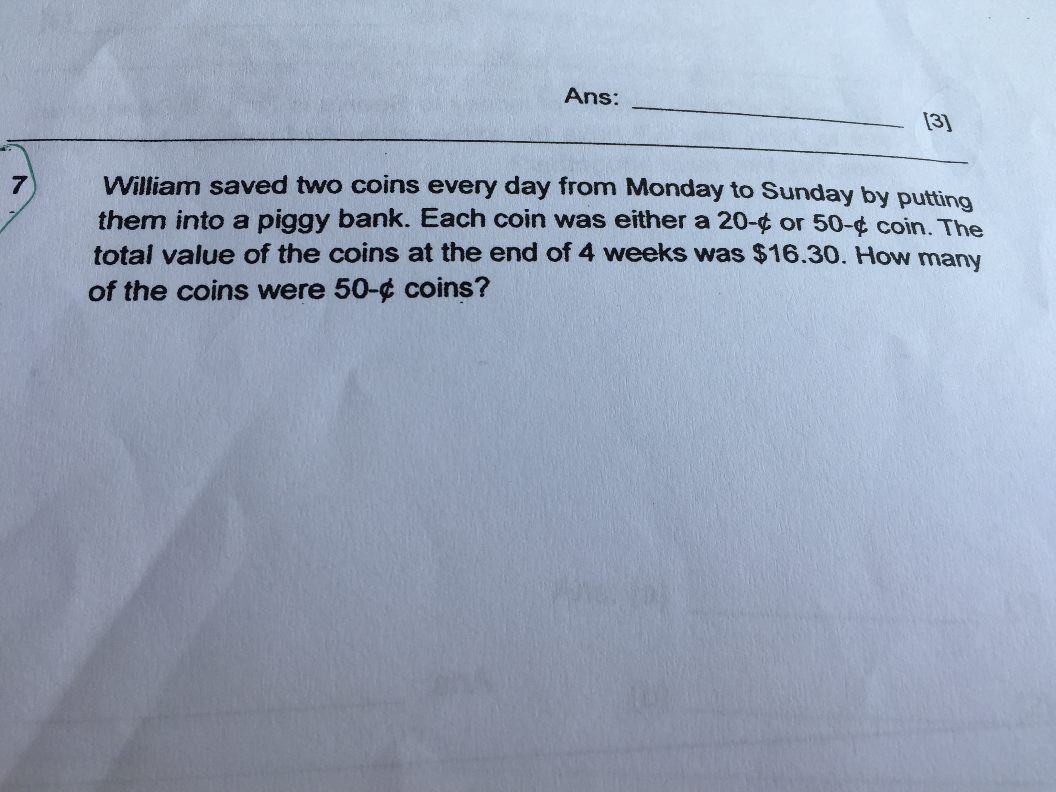# QuestionHi my solution is as follow \$16.30 divide by \$0.70 = 23 r 0.2. So my answer is 23 but answer given is 17. Pls assist. Thks

“Hi my working is as follow \$16.30 divide by \$0.70 = 23 R 0.2 so my answer is 23 but the answer given is 17. Can assist on this question. Thks ” I believe you assumed that William put in one 20c and 1 50c coin every day but this was not the case as the question did not state so. It could therefore be two 20c or 50c coins too.

The workings could be as follows :

4 weeks = 28 days
28 x 2 = 56 (coins)
56 x 0.20 = 11.20 (Assume all 56 are 20c coins)
16.30 – 11.20 = 5.10
0.50 – 0.20 = 0.30 (difference)
5.10/0.30 = 17 (50c coins)

Ans : 17 50c coins.

0 Replies 0 Likes